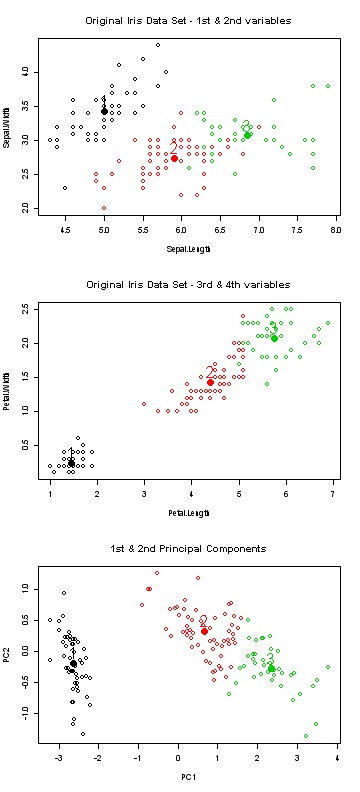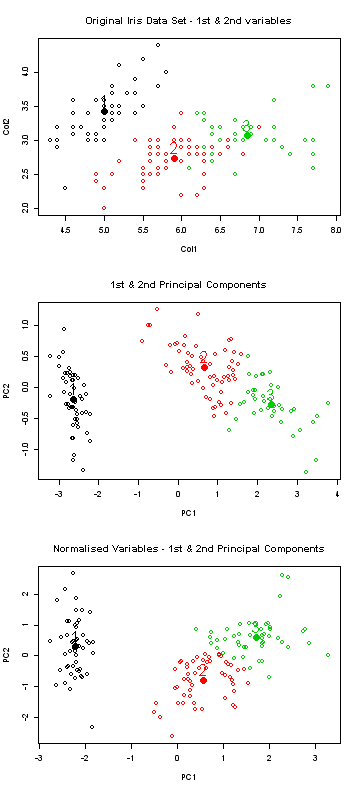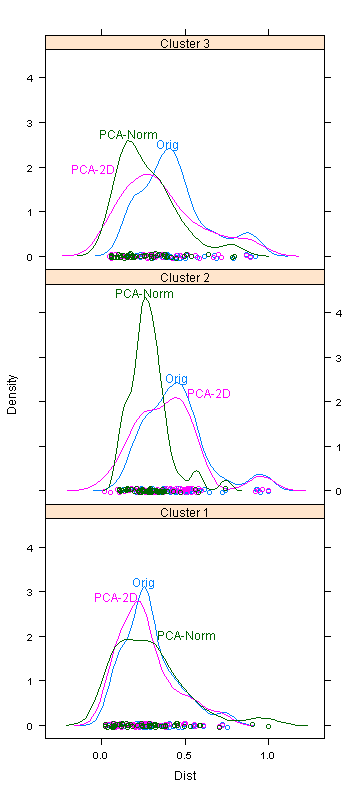## Tuesday, 7 January 2014

### Hybridisation of K-Means Clustering Analysis and Principal Component Analysis

Perhaps the most important issue when performing K-Means Clustering Analysis, or any clustering analysis for that matter, would be how well clusters are formed against the given variables within the data set. In some cases, outliers may need to be eliminated before performing clustering analysis as they sometimes interfere with forming best clusters.

This hybridisation technique aims to manipulate the data so that more distinct clusters are formed without losing any data points. Principal components are used in multidimensional scaling when performing clustering analysis. In this technique, the variables are normalised before principal components are derived. This has an effect of congregating data points into clusters more densely, hence separating clusters more distinctly.

The technique used in this blog modified the technique described in the below paper.
A hybridized K-means clustering approach for high dimensional dataset; Rajashree Dash, Debahuti Mishra, Amiya Kumar Rath, Milu Acharya; International Journal of Engineering, Science and Technology Vol. 2, No. 2, 2010, pp. 59-66; http://ijest-ng.com/ijest-ng-vol2-no2-pp59-66.pdf

To demonstrate the effect of this technique, iris data set was used.

Dat <- iris[, 1:4]
CTR <- aggregate(. ~ Species, iris, mean)

#clustering on the original data
km2 <- kmeans(Dat, centers = CTR[, 2:5], iter.max = 100)

#clustering on the principal components
PC <- princomp(Dat)
PC2 <- as.data.frame(PC\$score[, 1:2])
PC2\$Species <- iris\$Species
CTR_PC2 <- aggregate(. ~ Species, PC2, mean)
kmPC <- kmeans(PC2[, 1:2], centers = CTR_PC2[, 2:3], iter.max = 100)

The below graph compares clusters formed by the original data set to clusters formed against its principal components. It is clearly shown that clusters formed against principal components are better congregated and there are less overlaps between clusters in 2 dimensional display.

par(mfrow = c(3,1))
plot(Dat[, 1:2], col = km2\$cluster, main = "Original Iris Data Set - 1st & 2nd variables") points(km2\$centers[, 1:2], col = c(1:3), pch = 16, cex = 2)
text(km2\$centers[, 1:2], labels = c(1:3), col = c(1:3), pos = 3, cex = 2)

plot(Dat[, 3:4], col = km2\$cluster, main = "Original Iris Data Set - 3rd & 4th variables") points(km2\$centers[, 3:4], col = c(1:3), pch = 16, cex = 2)
text(km2\$centers[, 3:4], labels = c(1:3), col = c(1:3), pos = 3, cex = 2)

plot(PC2[, 1:2], col = kmPC\$cluster, xlab = "PC1", ylab = "PC2", main = "1st & 2nd Principal Components")
points(kmPC\$centers, col = c(1:3), pch = 16, cex = 2)
text(kmPC\$centers, labels = c(1:3), col = c(1:3), pos = 3, cex = 2)In this hybridisation technique, we are normalising the variables prior to deriving principal components as shown below. Then, only the principal components with respective eigen values greater than the average eigen values of all principal components are used in the clustering analysis.

V <- apply(Dat, 2, var) Input <- Dat[, which(V > 0)] Mean <- apply(Input, 2, mean) Sdev <- apply(Input, 2, sd) Adj.Data <- t(apply(Input, 1, function(x) (x - Mean))) Norm.Data <- t(apply(Adj.Data, 1, function(x) (x/Sdev))) Cov <- var(Norm.Data) Eig.Vec <- eigen(Cov) Featured <- Eig.Vec[][, 1:2] temp <- t(Featured) %*% t(Norm.Data) test1 <- t(temp) DatN <- cbind(as.data.frame(test1), Species = iris\$Species) CTR_PCN <- aggregate(. ~ Species, DatN, mean) kmPCN <- kmeans(test1, centers = CTR_PCN[, 2:3], iter.max = 100)

The below compares clusters formed by original variables, by principal components and by principal components of normalised variables. The main difference with normalisation is that the outliers become more distinct when clusters are formed in this example.

colnames(Dat) <- paste("Col", c(1:4), sep = "") Dat\$Species <- iris\$Species Dat\$Cluster <- km2\$cluster Dat\$Dist <- apply(Dat, 1, function(x) sqrt(sum((as.numeric(x[1:4]) - km2\$center[as.numeric(x), ])^2))) Dat\$Ind <- "Orig" colnames(PC2)[1:2] <- paste("Col", c(1:2), sep = "") PC2\$Cluster <- kmPC\$cluster PC2\$Dist <- apply(PC2, 1, function(x) sqrt(sum((as.numeric(x[1:2]) - kmPC\$center[as.numeric(x),])^2))) PC2\$Col3 <- NA PC2\$Col4 <- NA PC2\$Ind <- "PCA-2D" colnames(DatN)[1:2] <- paste("Col", c(1:2), sep = "") DatN\$Cluster <- kmPCN\$cluster DatN\$Dist <- apply(DatN, 1, function(x) sqrt(sum((as.numeric(x[1:2]) - kmPCN\$center[as.numeric(x),])^2))) DatN\$Col3 <- NA DatN\$Col4 <- NA DatN\$Ind <- "PCA-Norm" DatX <- rbind(Dat, PC2, DatN) DatX1 <- split(DatX, DatX\$Ind) DatX1 <- lapply(DatX1, function(x) { x\$Dist <- x\$Dist/max(x\$Dist) x })
DatX1 <- ldply(DatX1, data.frame)

par(mfrow = c(3, 1)) plot(Dat[, 1:2], col = km2\$cluster, main = "Original Iris Data Set - 1st & 2nd variables") points(km2\$centers[, 1:2], col = c(1:3), pch = 16, cex = 2) text(km2\$centers[, 1:2], labels = c(1:3), col = c(1:3), pos = 3, cex = 2)
plot(PC2[, 1:2], col = kmPC\$cluster, xlab = "PC1", ylab = "PC2", main = "1st & 2nd Principal Components") points(kmPC\$centers, col = c(1:3), pch = 16, cex = 2) text(kmPC\$centers, labels = c(1:3), col = c(1:3), pos = 3, cex = 2)
plot(DatN[, 1:2], col = kmPCN\$cluster, xlab = "PC1", ylab = "PC2", main = "Normalised Variables - 1st & 2nd Principal Components") points(kmPCN\$centers, col = c(1:3), pch = 16, cex = 2) text(kmPCN\$centers, labels = c(1:3), col = c(1:3), pos = 3, cex = 2)The below examines the density of each cluster when different methods are applied. It can be seen that normalised principal components generally forms denser clusters.

direct.label(densityplot(~Dist | paste("Cluster", Cluster, sep = " "), DatX1, groups = DatX1\$Ind,layout = c(3, 1)))